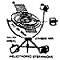Panagiotis Stefanides
 All triangles derive their origin from two triangles [ Pl.Ti. 53 D]. All triangles derive their origin from two triangles [ Pl.Ti. 53 D]. By *Panagiotis Chr. Stefanides ....all triangles derive their origin from two triangles, each having one angle right and the others acute....These we lay down as the principles of fire and all the other bodies...Pl.Ti. 53 D. One of these two triangles is of course  the 45/45 deg [tan 45 equals 1] orthogonal triangle and my interpretation of the other scalene orthogonal is that whose angle between hypotenuse and the horizontal smaller has as tangent the sqrt[Φ], contrary to the currently accepted interpretation of the  30/60 deg scalene orthogonal one. We consider the following: sqrt[Φ]= sqrt[[sqrt(5) + 1 ]/2] = 1.27201965…= T , with corresponding angle θ, so that: θ = Arctan(T) = 51.82729238… deg. An implementation of the relationships involving the two triangles [angles tan(45) deg. and tan(θ)], is: Sqrt[ [tan(45)+sqrt[5*tan(45)]*cos(60)] = tan(51.82729238…)=1.27201965..=T =sqrt[Φ], or  [1+sqrt(5)] / 2] = Φ, where, Φ = 1.618033989… , with corresponding angle φ, so that:   φ = Arctan(Φ)= Arctan(T^2) = 58.28252559…deg.                  θ  = Arctan(T)                θ  = 51.82729238… deg., and                Tan [θ] = T ,                 Tan [φ] = T^2= 1.618033989..=Φ                [Tan [θ]]^2 = Tan [φ], or                Tan [θ ]= sqrt[Tan [φ]]                θ = Arctan[sqrt[Tan[φ]]]                Angle 60 deg.  [the constituent equilateral triangle angle[Pl.Ti.54 E],                formed by 6 scalene orthogonal triangles of the 30/60 deg. form] is                    related to:  2 =[sqrt(5) +1]/ Φ, as 60 deg. =Arcos[1/2]. Since: θ = Arctan[sqrt[Tan [φ]]], i.e angle θ  is related to angle  φ, and Since: Sqrt[ [tan(45)+sqrt[5*tan(45)]*cos(60)] =tan(51.82729238…)=1.27201965..=T =sqrt[Φ] = tan[ θ], then angles θ, and 45, are related also to angle 60 deg.   Logarithmic Relationships: According to my manipulation of basic logarithms [Using the known relationship for finding logarithm to base 2 of a NUMBER as the ratio : Ln[NUMBER] / Ln , Ln being the Natural Logarithm [base e], all [positive Real] numbers [ or angles ] are related to angles Arctan[T] =51.82729237..deg., and  45 deg:  [tan(45)+[sqrt(5)+1] / e^{[ln[ln[NUMBER]] ]- ln[ln[sqrt[NUMBER]]]}]^0.25 = = 1.27201965..=T =sqrt[Φ]. Examples: [tan(45)+[sqrt(5)+1] / e^{[ln[ln] ]- ln[ln[sqrt]]}]^0.25 = 1.27201965..=T =sqrt[Φ] [tan(45)+[sqrt(5)+1] / e^{[ln[ln[0.1]] ]- ln[ln[sqrt[0.1]]]}]^0.25= 1.27201965..   Number: 2=2*tan(45)=1/[cos(60)], Logarithmic Relationship: The Logarithmic form involving number 2, [giving the relationship for the 30/60 deg. orthogonal triangle, from which the equilateral triangle is produced], is as follows:  Ln[Ln[+positive real NUMBER or angle]] – Ln[Ln[sqrt(+positive real NUMBER or angle )]] = =0.693147181.. Ln(2) = 0.693147181..=Ln[1/[cos(60)]], = Ln [[ sqrt(5*tan(45) +tan(45)]/T^2]. e^[0.693147181.]=2 Example: Ln[Ln] – Ln[Ln[sqrt(16)]] = Ln(2)= Ln [[ (5*tan(45) +tan(45)]/T^2] = 0.693147181...   A Great Pyramid Model [G.P.] relationship with the 5 solids.  A Great Pyramid Model [G.P.],  its structure being based upon this theory of the proposed new form of the “Most Beautiful Triangle” relates θ and 45 angles triangles with angles φ, found on each of the isosceli triangular faces of the G.P. By cutting in half one of this face [by a vertical from its vertex perpendicular to its base] we get two orthogonal triangles, bearing angle φ  [and 90- φ]. Adding them along their hypotenuse we get a parallelogramme plane. Placing three such planes each perpendicular to each other, automatically we get 20 equilateral triangles[60 deg.] defined by the vertices of the such structured planes. So we constructed the icosahedron via this process. In a similar way we do so for the structure of Ether [Quintessence] or  dodecahedron [ since we find Φ^2 = 2.618033989..or T^4, in  a selective section of this structure, and , also angle φ]. The 45 deg. [ Arcsin[1/sqrt(2) = Arcos [1/sqrt(2)]  structures the square and the cube, which , within cube relates to sqrt(3), i.e. angle: arcsin[1/sqrt(3)]=35.26438968..deg. and 90-35.26438968.. = 54.73561032..deg., both of  which found within selective sections of the tetrahedron and the octahedron. Theory of Lines Building the World Solids are formed by triangle surfaces originating from the two triangles the special scalene orthogonal and the isosceles orthogonal. Triangles sides are formed from lines based on kinds of  4 lines in geometric ratio to each other T, i.e. lengths corresponding to : T^3, T^2, T^1 and 1. The special scalene orthogonal is formed from the lines T^3, T^2, T^1, the isosceles orthogonal from 1, forming the square with sides 1. A second triangle similar to the special scalene is formed with sides T^2, T^1, and 1. This is constituent orthogonal triangle of the first. Two pairs of such triangles joined together build[SOMATOIDES] the first solid constituent of a G.P. Model and thus of the 5 Platonic or Euclidean Solids. © Copyright 1986 - 2011 Panagiotis Chr. Stefanides *Eur Ing Panagiotis Stefanides BSc(Eng) Lon(Hons)  MSc(Eng)NTUA TEE CEng MIET Emeritus Honoured Member of the Technical Chamber of Greece     [TEE] Ex Hellenic Aerospace Ind S.A.,  R and D Lead Engineer and Directorate Aircraft Engineer Manufacturing  Engineering Methods’ Superintendent. e: Budapest: “Golden Root Symmetries of Geometric Forms”    http://www.stefanides.gr/Html/GOLDEN_ROOT_SYMMETRIES.htm  ,  [Book  Published 2010 ] Publication: “Golden Root Symmetries of Geometric Forms” Symmetry: Culture and Science, The journal of the Symmetrion ,Volume 17, Numbers 1-2, 2006, pp 97-111. Award: Archimedes Silver Medal for the Invention of a Solar Locating and Tracking  System, by the Hellenic Society of Research and Inventions, Athens Hilton 4 Feb 1983  [“Hliotropio Stefanides” Competed  in Create the Future Design Contest 2011, of the NASA Tech Briefs :   http://contest.techbriefs.com/sustainable-technologies-2011/1179  ]. © Copyright 1986 - 2011 P. C. Stefanides.